# 经验干货：使用tf.py_func函数增加Tensorflow程序的灵活性

不知不觉，笔者接触Tensorflow也满一年了。在这一年当中，笔者对Tensorflow的了解程度也逐渐加深。相比笔者接触的第一个深度学习框架Caffe而言，笔者认为Tensorflow更适合科研一些，网络搭建与算法设置的自由度也更大，使用Tensorflow实现自己的算法也更迅速。

但是，笔者认为Tensorflow还是有不足的地方。第一体现在Tensorflow的数据机制，由于tensor只是占位符，在没有用tf.Session().run接口填充值之前是没有实际值的。因此，在网络搭建的时候，是不能对tensor进行判值操作的，即不能插入if...else...之类的代码。第二，相较于numpy array，Tensorflow中对tensor的操作接口灵活性并没有那么高，使得Tensorflow的灵活性减弱。

在笔者使用Tensorflow的一年中积累的编程经验来看，扩展Tensorflow程序的灵活性，有一个重要的手段，就是使用tf.py_func接口。笔者先对这个接口做出解析：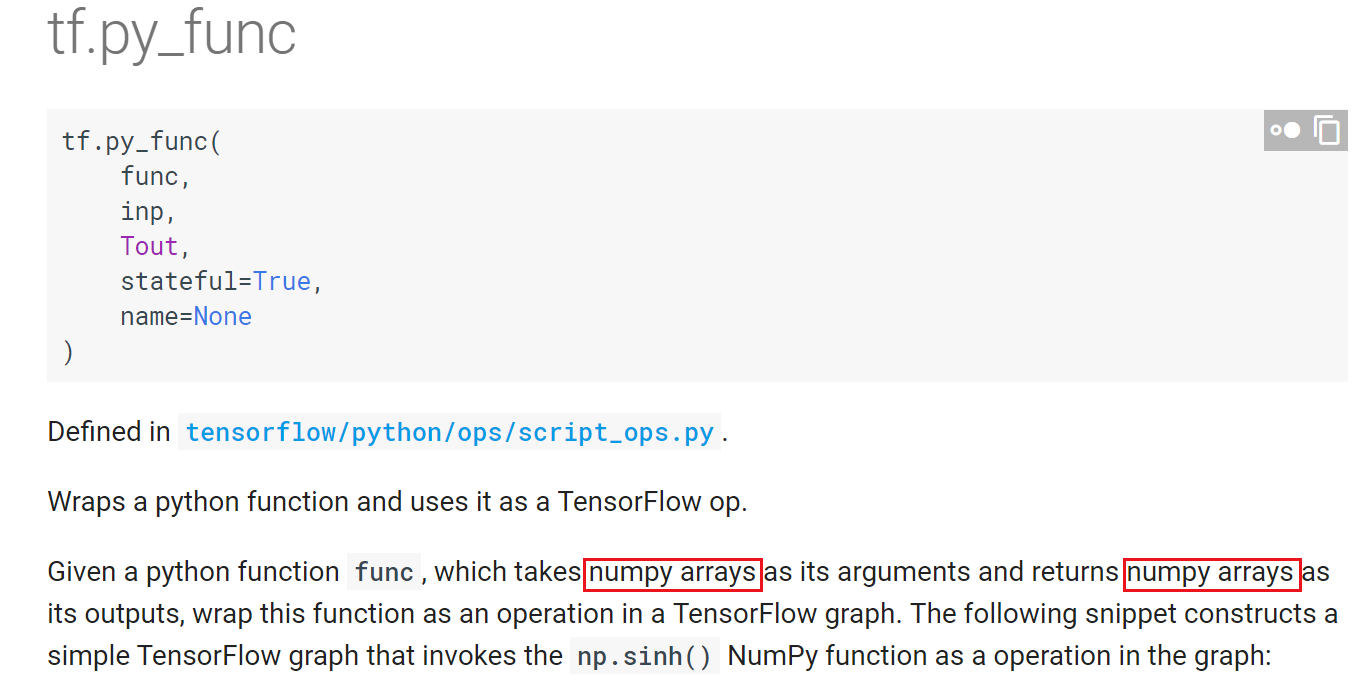在上图中，我们看到，tf.py_func的核心是一个func函数(由用户自己定义)，该函数接收numpy array作为输入，并返回numpy array类型的输出。看到这里，大家应该能够明白为什么建议使用py_func，因为在func函数中，可以对转化成numpy array的tensor进行np.运算，这就大大扩展了程序的灵活性。

然后，我们来看看tf.py_func接受什么参数：在使用tf.py_func的过程中，主要核心是使用前三个参数。

第一个参数func，也是最重要的，是一个用户自定制的函数，输入numpy array，输出也是numpy array，在该函数中，可以自由使用np.操作。

第二个参数inp，是func函数接收的输入，是一个列表

第三个参数Tout，指定了func函数返回的numpy array转化成tensor后的格式，如果是返回个值，就是一个列表或元组；如果只有个返回值，就是一个单独的dtype类型(当然也可以用列表括起来)。

最后来看看tf.py_func的输出：输出是一个tensor列表或单个tensor。

到这里，tf.py_func的原理也就逐渐明晰了。首先，tf.py_func接收的是tensor，然后将其转化为numpy array送入func函数，最后再将func函数输出的numpy array转化为tensor返回。

在使用过程中，有两个需要注意的地方，第一就是func函数的返回值类型一定要和Tout指定的tensor类型一致。第二就是，如下图所示，tf.py_func中的func是脱离Graph的。在func中不能定义可训练的参数参与网络训练(反传)。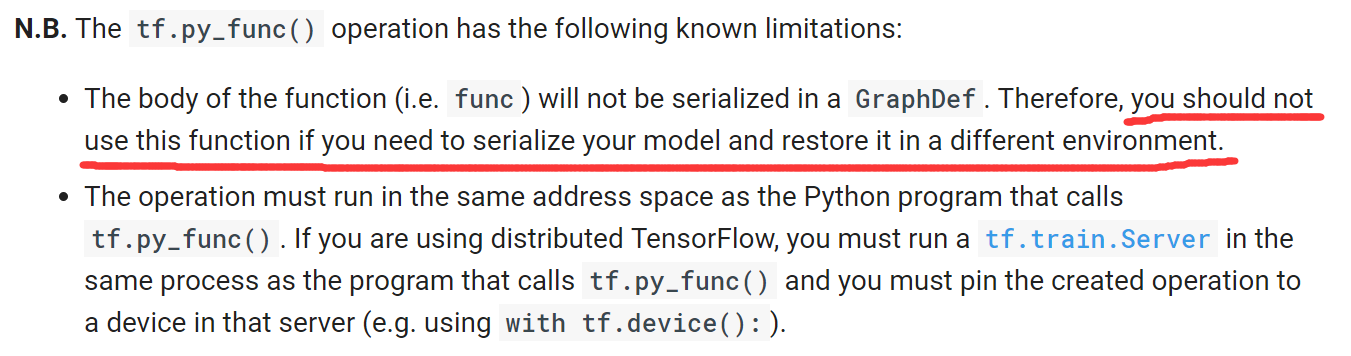上面就解析了tf.py_func的使用方法和原理。下面笔者举几个例子，一是向大家展示tf.py_func带来的灵活性，二是通过笔者的亲身体会说明一下如何使用tf.py_func完成一些Tensorflow基础编程中较难的任务。

1) tf.py_func在Faster R-CNN中的接口中的使用。

在目标检测算法Faster R-CNN中，需要计算各种ground truth，接口比较复杂。因此，使用tf.py_func是一个比较好的途径。对于tf.py_func的使用，可以参见计算RPN的ground truth计算proposals的ground truth时的使用方法。可以看到，都是将tensor转化成numpy array，再使用np.操作完成复杂运算。

下面笔者来举两个小例子，说明一下tf.py_func的强大功能。

2) 使用tf.py_func获得未知tensor维度。

大家知道，我们在做数据占位的时候，可能会传入"None"，即不知道数据的该维大小，取决于feed_dict中的实际值。可是，在运算中，要使用到数据的该维大小时应该怎么办呢？比如下面这个例子：

import tensorflow as tf
import numpy as np

def main():
a = tf.placeholder(tf.float32, shape=[1, 2], name = "tensor_a")
b = tf.placeholder(tf.float32, shape=[None, 2], name = "tensor_b")
tile_a = tf.tile(a, [b.get_shape(), 1])
sess = tf.Session()
array_a = np.array([[1., 2.]])
array_b = np.array([[3., 4.],[5., 6.],[7., 8.]])
feed_dict = {a: array_a, b: array_b}
tile_a_value = sess.run(tile_a, feed_dict = feed_dict)
print(tile_a_value)

if __name__ == '__main__':
main()

如上代码所示，要完成一个很简单的功能，就是扩张tensor a，将其的维度变成和tensor b一样，可是tensor b的维度暂时未知。我们来看看，执行上述程序能得到什么结果：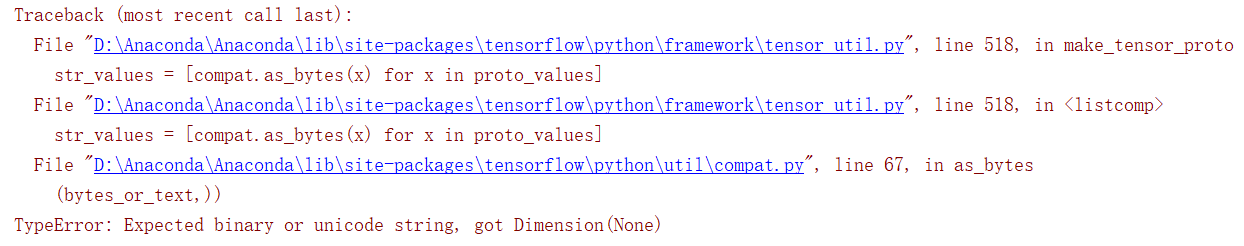可以看到，由于tensor b第一个维度未知，因此在给tile_a分配存储空间时报错，提示不能有None存在。

如何解决这个问题？稍微改写一下上述代码，让tensor扩张在tf.py_func中执行：

import tensorflow as tf
import numpy as np
from py_func_1 import *

def main():
a = tf.placeholder(tf.float32, shape=[1, 2], name = "tensor_a")
b = tf.placeholder(tf.float32, shape=[None, 2], name = "tensor_b")
tile_a = tile_tensor(a, b)
sess = tf.Session()
array_a = np.array([[1., 2.]])
array_b = np.array([[3., 4.],[5., 6.],[7., 8.]])
feed_dict = {a: array_a, b: array_b}
tile_a_value = sess.run(tile_a, feed_dict = feed_dict)
print(tile_a_value)

if __name__ == '__main__':
main()

在上面的代码中，tensor扩张在tile_tensor这个函数中执行。该函数定义在py_func_1.py文件中，下面是py_func_1.py的代码：

import tensorflow as tf
import numpy as np

def tile_tensor(tensor_a, tensor_b):
tile_tensor_a = tf.py_func(_tile_tensor, [tensor_a, tensor_b], tf.float32)
return tile_tensor_a

def _tile_tensor(a, b):
tile_a = np.tile(a, (b.shape, 1))
return tile_a
大家可以看到，使用了tf.py_func接口，参数func就是_tile_tensor函数。在 _tile_tensor函数中，将a扩张了，执行一下修改后的main函数，输出结果：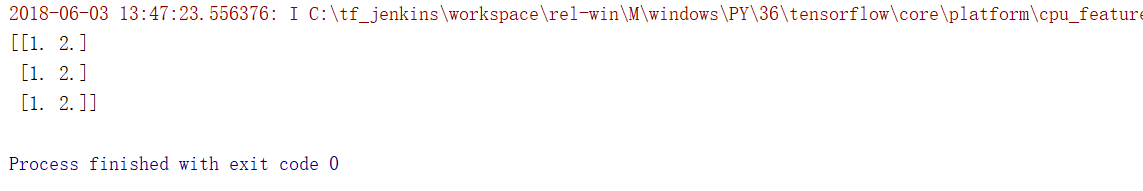大家可以看到，在tile_tensor函数中，tensor a在tensor b的维度未知的情况下，根据tensor b的实际维度([3, 2])将其扩张了。并返回了一个tensor类型的tile_a。

3) 在tf.py_func中对tensor的值作出判断。

笔者在之前的博客中提到过，在tf.Session().run之前，是不能对Tensor的值做出判断的。比如，我们想根据tensor a的值对tensor b做出扩张：

import tensorflow as tf
import numpy as np

def main():
a = tf.placeholder(tf.float32, shape=, name = "tensor_a")
b = tf.placeholder(tf.float32, shape=[1, 2], name = "tensor_b")
tile_b = b
if a==1.:
tile_b = tf.tile(b, [4, 1])
sess = tf.Session()
array_a = np.array([1.])
array_b = np.array([[2., 3.]])
feed_dict = {a: array_a, b: array_b}
tile_b_value = sess.run(tile_b, feed_dict = feed_dict)
print(tile_b_value)

if __name__ == '__main__':
main()

如果a的值为1.0，那么就将tensor b扩张四倍。我们执行一下上述代码看看结果：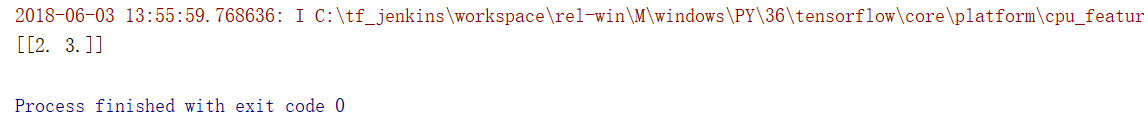大家可以看到，由于在if语句执行时，tensor a里面是空的。因此，不会执行if中的语句。尽管在feed_dict中a被填充了1.0，并且程序不报错，可是没有达到预想的目标。

如何解决这个问题？稍微改写一下上述代码，让判值进行tensor扩张在tf.py_func中执行：

import tensorflow as tf
import numpy as np
from py_func_2 import *

def main():
a = tf.placeholder(tf.float32, shape=, name = "tensor_a")
b = tf.placeholder(tf.float32, shape=[1, 2], name = "tensor_b")
tile_tensor_b = tile_b(a, b)
sess = tf.Session()
array_a = np.array([1.])
array_b = np.array([[2., 3.]])
feed_dict = {a: array_a, b: array_b}
tile_b_value = sess.run(tile_tensor_b, feed_dict = feed_dict)
print(tile_b_value)

if __name__ == '__main__':
main()

大家可以看到，在py_func_2.py中的tile_b函数中，对tensor b进行了判值扩张。py_func_2.py代码如下所示：

import tensorflow as tf
import numpy as np

def tile_b(tensor_a, tensor_b):
tile_tensor_b = tf.py_func(_tile_b, [tensor_a, tensor_b], tf.float32)
return tile_tensor_b

def _tile_b(a, b):
if a==1.:
tile_b = np.tile(b, (4, 1))
else:
tile_b = b
return tile_b

大家可以看到，在tile_b函数中有一个tf.py_func函数，其中的func参数便是_tile_b函数。在_tile_b函数中，根据a的值对b进行了扩张。我们来运行一下main函数，输出结果：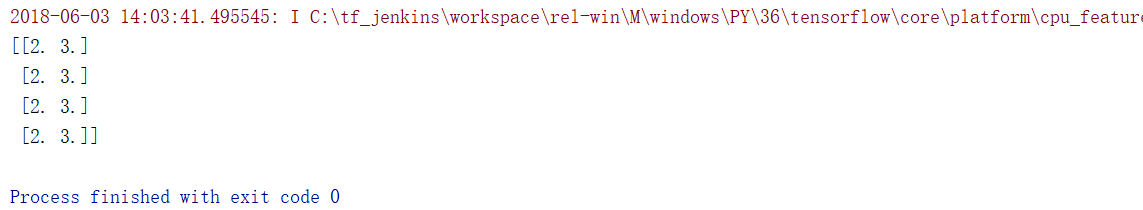tensor b得到了扩张！

大家可以看到，在tensor输入进tf.py_func并转化成numpy array后，判值操作就有效了。

通过上面的三个例子，笔者向大家揭示了tf.py_func函数中的神奇之处。大家可以看到，在实际使用中，将tensor转化为numpy array后，能够执行更灵活的操作，达到更多的目标。总而言之，tf.py_func是一个很强大的接口，也希望大家能在Tensorflow程序中灵活运用。

欢迎阅读笔者后续博客，各位读者朋友的支持与鼓励是我最大的动力！

written by jiong

07-042909
07-02326905-271718
06-075624
11-292037
02-144821
11-137933
05-06729
08-112771
10-051627